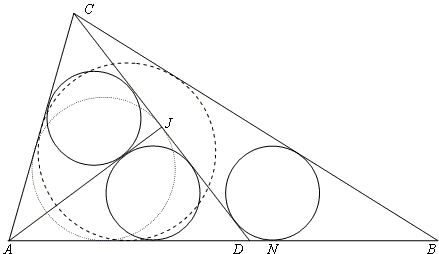# A Trigonometric Solution to a Difficult Sangaku Problem

### Michel Cabart Feb. 25, 2010

PROBLEM. In ΔABC, AB = BC. If one chooses D on AB and J on CD such that AJ ⊥ CD and the incircles of ΔACJ, ΔADJ, and ΔBCD all have radius r, then r = AJ/4.SOLUTION

Let's say ∠ADC = x, AD = 1 and tan(x/2) = t. We calculate ρ, the radius of the incircle of ΔADJ by a known formula for the radius of the incircle:

with: tan(∠ADJ) = t, tan(∠DAJ) = tan(45° - x/2) = (1 - t)/(1 + t). So that

ρ = t(1 - t)/(t² + 1) .

Similarly r2 = CD·tan(∠CDO2)·tan(∠DCO2) / (tan(∠CDO2) + tan(∠DCO2)),

with CD = 2 cos(x) = 2 (1 - t²)/(1 + t²). Further,

tan(∠CDO2 = tan(90° - x/2) = 1/t,
tan DCO2 = tan (90° - 3x/2) = (1 - 3t2)/(3t - t3),

so that r2 = ½(1 - 3t²)/t(1 + t²).

Equating ρ and r2 yields the equation:

2t3 - 5t2 + 1 = (2t - 1)·(t2 - 2t - 1) = 0.

The second (quadratic) factor cannot be zero as it would lead to t = 1 ± 2 and x = 135°.

The solution is then t = 1/2, leading to ρ = r2 = 1/5, AJ = sin(x) = 4/5, JD = cos(x) = 3/5.## Sangaku